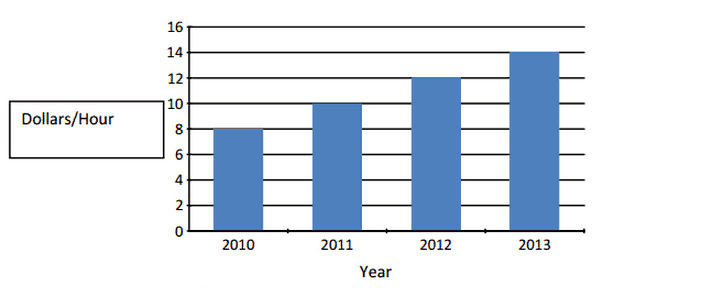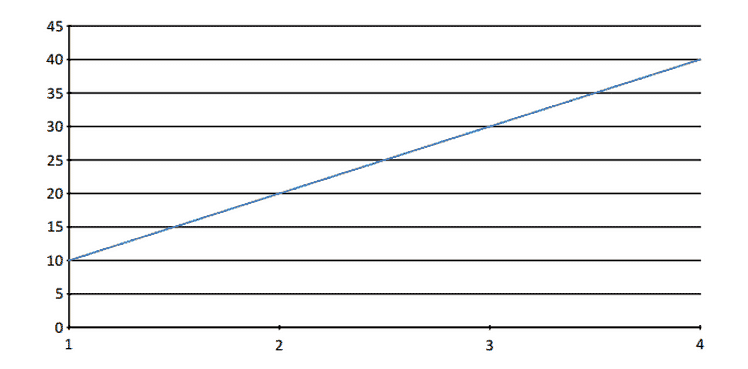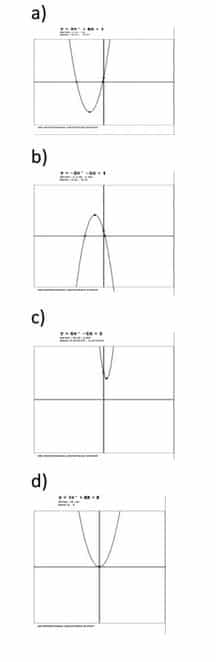# GED Math Practice Test 1 | Free GED Study Guide: A Faster Way to Pass

GED Math Practice Test 1 to improve your GED Scores. Our free GED sample questions will help you pass your GED exams. Try our Practice Tests.

We have GED Math Video Lessons

## Math Practice Questions – GED Free Online Practice Tests

GED Math Practice Test 1 to improve your GED scores. Try our practice questions to prepare for all subjects and pass your GED. Math practice questions are based on the latest GED exam that will help you ace your GED. It’s never been late to start preparing for the GED test. So, don’t wait anymore and start with our free practice tests for all subjects.

This practice test covers all the relevant topics of the original GED math test. Each question will help you learn and improve your knowledge.

Check our Free GED Math Practice Test HERE

The GED® Math test cover topics like:

• Number operations & number sense = 20-30%
• Measurement & geometry = 20-30%
• Data analysis, statistics, & probability = 20-30%
• Algebra, functions, & patterns = 20-30%

The GED Math test has 46 questions. You need to finish the test in 115 minutes or less. You need to get a score of 145 to pass this section. A calculator and formula and symbols sheet will be provided.

# GED Math Practice Questions

GED Math Practice Questions. No signup. Free Studying tools from flash cards, practice questions, to mini lessons. Everything you need to study for the GED. All GED practice tests are based on recent GED Exam questions. Start today and secure your GED at first attempt.

## Math Practice Questions – GED Free Online Practice Tests

GED free online practice test for math to improve your GED scores. Try our practice questions to prepare for all subjects and pass your GED. Math practice questions are based on latest GED exam that will help you ace your GED. It’s never been late to start preparing for the GED test. So, don’t wait anymore and start with our free practice tests for all subjects.

This practice test covers all the relevant topics of the original GED math test. Each question will help you learn and improve your knowledge.

The GED® Math test cover topics like:

• Number operations & number sense = 20-30%
• Measurement & geometry = 20-30%
• Data analysis, statistics, & probability = 20-30%
• Algebra, functions, & patterns = 20-30%

The GED Math test has 46 questions. You need to finish the test in 115 minutes or less. You need to get a score of 145 to pass this section. A calculator and formula and symbols sheet will be provided.

Question 1 of 25 (click ‘Next Page’ at the bottom right of the page to go on to the next question.)

4 % Complete

A. \$27.63
B. \$30.00
C. \$17.50
D. \$37.38

Question 2 of 25

8 % Complete

A. 4(1/3)
B. 64
C. 64(1/3)
D. 464

Question 3 of 25

12 % Complete

A. 1/4
B. 1/8
C. 1/2
D. 10/16

Question 4 of 25

16 % Complete

A. 2a+1+12a
B. 6a+3+4a
C. 10a+3
D. 7a+8a2

Question 5 of 25

20 % Complete

A. P= 15K-10K
B. P=5K-10K
C. P=15K-5K
D. P=5K-5K

Question 6 of 25

24 % Complete

A. 341 inches
B. 314 inches
C. 25 inches
D. 300 inches

Question 7 of 25

28 % Complete

y= 10x2-7x-12

A. 57
B. 21
C. 91
D. 55

## Question 8 | GED Math Practice Test 1

Question 8 of 25

32 % Complete

### Using the graph below, calculate how much money you would earn per hour in 2016? (hint: y=mx+b)A. 20/Hr
B. 16/Hr
C. 10/Hr
D. 22/Hr

Question 9 of 25

36 % Complete

## Question 10 | GED Math Practice Test 1

Question 10 of 25

40 % Complete

A. X2-8X-6
B. 2X2-8X+6
C. 2X2+8X+6
D. X2+4X+3

## Question 11 | GED Math Practice Test 1

Question 11 of 25

44 % Complete

A. \$10.00
B. \$35.00
C. \$8.00
D. \$7.00

## Question 12 | GED Math Practice Test 1

Question 12 of 25

48 % Complete

A. 70
B. 63
C. 67
D. 30

## Question 13 | GED Math Practice Test 1

Question 13 of 25

52 % Complete

A. 9X5+4X2+4X+7
B. 7X3+6X2+4X+7
C. 9X3+6X2+8X+2
D. 7X3+4X4+3X2+7

## Question 14 | GED Math Practice Test 1

Question 14 of 25

56 % Complete

### Penny went for a four mile run as shown by the graph below. (The Y-axis for the graph is time in minutes, while the X axis is distance in miles) Victor ran a different route than Penny. He ran 10 miles in 1 ½ hours. Who ran at faster speed?A. Victor
B. Penny
C. Neither. They both ran at the same speed.

## Question 15 | GED Math Practice Test 1

Question 15 of 25

60 % Complete

## Question 16 | GED Math Practice Test 1

Question 16 of 25

64 % Complete

### Alice needs 36 inches of ribbon to complete her art project. At the store she buys four and a half feet of ribbon. How many inches of ribbon is Alice going to have to cut off?

(hint: There are 12 inches in one foot)

A. 36 inches
B. 24 inches
C. 18 inches
D. 5 inches

## Question 17 | GED Math Practice Test 1

Question 17 of 25

68 % Complete

## Question 18 | GED Math Practice Test 1

Question 18 of 25

72 % Complete

## Question 19 | GED Math Practice Test 1

Question 19 of 25

76 % Complete

A. \$1.60
B. \$4.00
C. \$10.00
D. \$3.00

## Question 20 | GED Math Practice Test 1

Question 20 of 25

80 % Complete

## Question 21 | GED Math Practice Test 1

Question 21 of 25

84 % Complete

## Question 22 | GED Math Practice Test 1

Question 22 of 25

88 % Complete

### Find the surface area of a cone whose height is 5m and radius is 6m.

Hint: You will need to use the following equations:
Height = h
Base Area= πr2
Slant Height = ℓ | h2+r2=ℓ2
Lateral Area: πℓr
Surface Area: Base Area+ Lateral Area

## Question 23 | GED Math Practice Test

Question 23 of 25

92 % Complete

### Amy is selling turtles for \$3.00 and rabbits for \$5.00. On Monday, she sold 28 animals for \$110.00. How many turtles and how many were rabbits? (hint: you must write two separate equations to later merge to solve this)

A. 25 turtles, 5 rabbits
B. 12 rabbits, 16 turtles
C. 8 turtles, 20 rabbits
D. 13 rabbits, 15 turtles

## Question 24 | GED Math Practice Test 1

Question 24 of 25

96 % Complete

### Which of the following graphs best represents this equation: y=2×2+8x+1 ?## Question 25 | GED Math Practice Test 1

Question 25 of 25

100 % Complete

A. 1/13
B. 1/2
C. 1/26
D. 1/8